作者：小傅哥 博客：https://bugstack.cn

## 一、前言

1. 数据插入流程和源码分析
2. 链表树化以及树转链表
3. 遍历过程中的无序Set的核心知识

🕵注意： 建议阅读上一篇后，再阅读本篇文章《HashMap核心知识，扰动函数、负载因子、扩容链表拆分，深度学习》

## 二、HashMap源码分析

### 1. 插入

#### 1.1 疑问点&考题

1. 如果出现哈希值计算的下标碰撞了怎么办？
2. 如果碰撞了是扩容数组还是把值存成链表结构，让一个节点有多个值存放呢？
3. 如果存放的数据的链表过长，就失去了散列表的性能了，怎么办呢？
4. 如果想解决链表过长，什么时候使用树结构呢，使用哪种树呢？

#### 1.2 插入流程和源码分析

HashMap插入数据流程图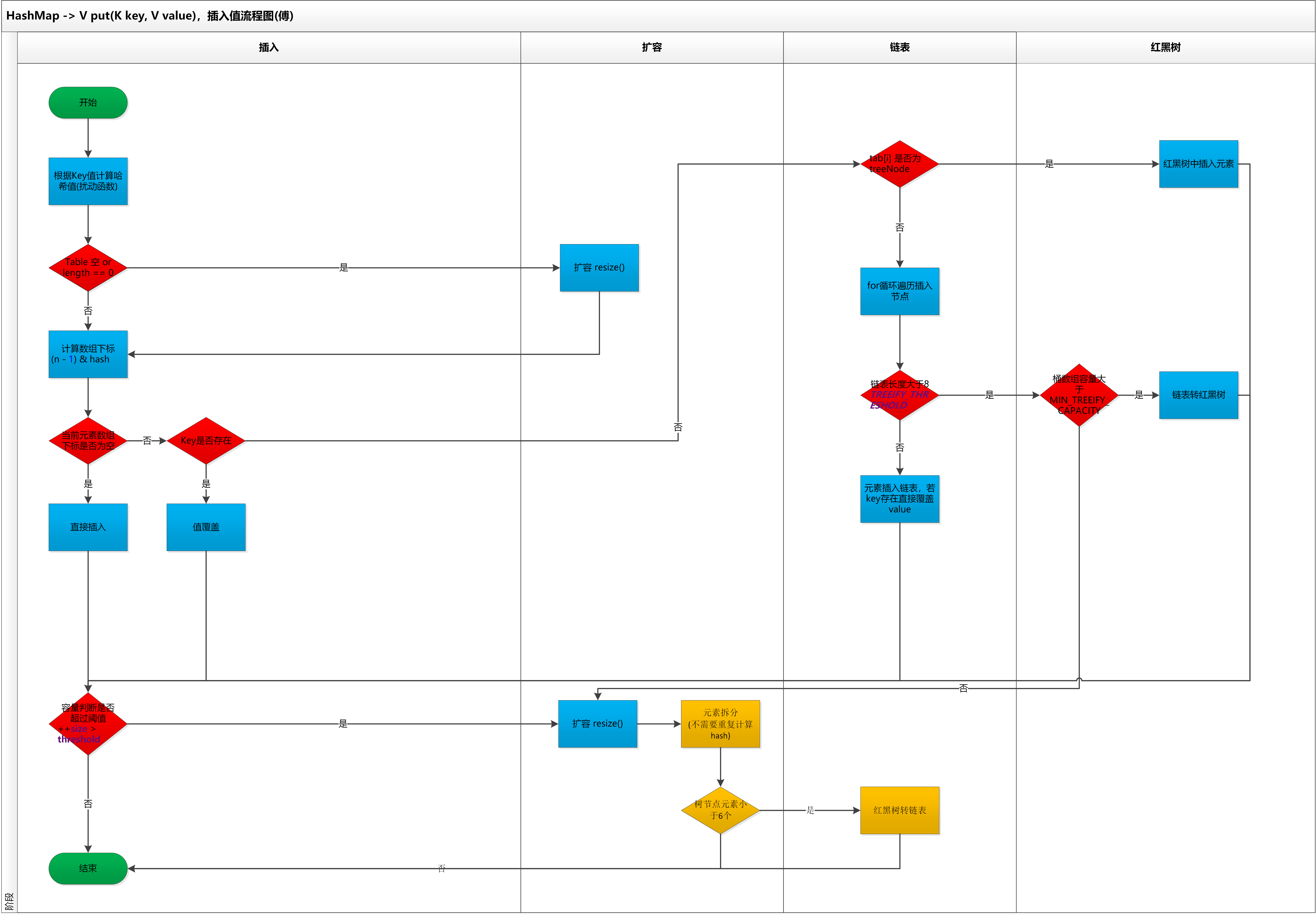visio原版流程图，可以通过关注公众号：bugstack虫洞栈，进行下载

1. 首先进行哈希值的扰动，获取一个新的哈希值。`(key == null) ? 0 : (h = key.hashCode()) ^ (h >>> 16);`

2. 判断tab是否位空或者长度为0，如果是则进行扩容操作。

``````if ((tab = table) == null || (n = tab.length) == 0)
n = (tab = resize()).length;``````
3. 根据哈希值计算下标，如果对应小标正好没有存放数据，则直接插入即可否则需要覆盖。`tab[i = (n - 1) & hash])`

4. 判断tab[i]是否为树节点，否则向链表中插入数据，是则向树中插入节点。

5. 如果链表中插入节点的时候，链表长度大于等于8，则需要把链表转换为红黑树。`treeifyBin(tab, hash);`

6. 最后所有元素处理完成后，判断是否超过阈值；`threshold`，超过则扩容。

7. `treeifyBin`,是一个链表转树的方法，但不是所有的链表长度为8后都会转成树，还需要判断存放key值的数组桶长度是否小于64 `MIN_TREEIFY_CAPACITY`。如果小于则需要扩容，扩容后链表上的数据会被拆分散列的相应的桶节点上，也就把链表长度缩短了。

JDK1.8 HashMap的put方法源码如下:

``````public V put(K key, V value) {
return putVal(hash(key), key, value, false, true);
}

final V putVal(int hash, K key, V value, boolean onlyIfAbsent,
boolean evict) {
Node<K,V>[] tab; Node<K,V> p; int n, i;
// 初始化桶数组 table，table 被延迟到插入新数据时再进行初始化
if ((tab = table) == null || (n = tab.length) == 0)
n = (tab = resize()).length;
// 如果桶中不包含键值对节点引用，则将新键值对节点的引用存入桶中即可
if ((p = tab[i = (n - 1) & hash]) == null)
tab[i] = newNode(hash, key, value, null);
else {
Node<K,V> e; K k;
// 如果键的值以及节点 hash 等于链表中的第一个键值对节点时，则将 e 指向该键值对
if (p.hash == hash &&
((k = p.key) == key || (key != null && key.equals(k))))
e = p;

// 如果桶中的引用类型为 TreeNode，则调用红黑树的插入方法
else if (p instanceof TreeNode)
e = ((TreeNode<K,V>)p).putTreeVal(this, tab, hash, key, value);
else {
// 对链表进行遍历，并统计链表长度
for (int binCount = 0; ; ++binCount) {
// 链表中不包含要插入的键值对节点时，则将该节点接在链表的最后
if ((e = p.next) == null) {
p.next = newNode(hash, key, value, null);
// 如果链表长度大于或等于树化阈值，则进行树化操作
if (binCount >= TREEIFY_THRESHOLD - 1) // -1 for 1st
treeifyBin(tab, hash);
break;
}

// 条件为 true，表示当前链表包含要插入的键值对，终止遍历
if (e.hash == hash &&
((k = e.key) == key || (key != null && key.equals(k))))
break;
p = e;
}
}

// 判断要插入的键值对是否存在 HashMap 中
if (e != null) { // existing mapping for key
V oldValue = e.value;
// onlyIfAbsent 表示是否仅在 oldValue 为 null 的情况下更新键值对的值
if (!onlyIfAbsent || oldValue == null)
e.value = value;
afterNodeAccess(e);
return oldValue;
}
}
++modCount;
// 键值对数量超过阈值时，则进行扩容
if (++size > threshold)
resize();
afterNodeInsertion(evict);
return null;
}``````

#### 1.3 扩容机制

HashMap是基于数组+链表和红黑树实现的，但用于存放key值得的数组桶的长度是固定的，由初始化决定。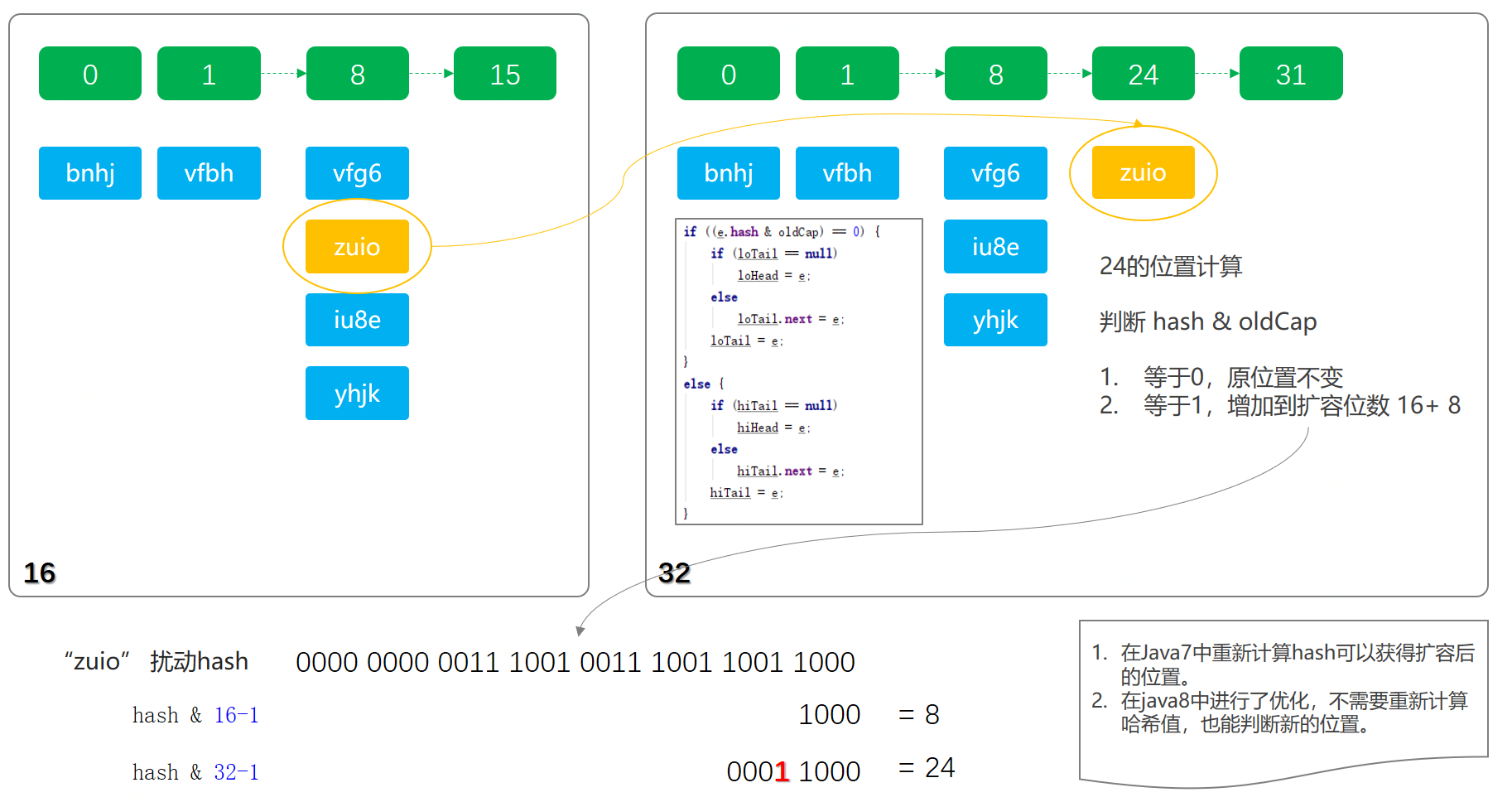``````final Node<K,V>[] resize() {
Node<K,V>[] oldTab = table;
int oldCap = (oldTab == null) ? 0 : oldTab.length;
int oldThr = threshold;
int newCap, newThr = 0;
// Cap 是 capacity 的缩写，容量。如果容量不为空，则说明已经初始化。
if (oldCap > 0) {
// 如果容量达到最大1 << 30则不再扩容
if (oldCap >= MAXIMUM_CAPACITY) {
threshold = Integer.MAX_VALUE;
return oldTab;
}

// 按旧容量和阀值的2倍计算新容量和阀值
else if ((newCap = oldCap << 1) < MAXIMUM_CAPACITY &&
oldCap >= DEFAULT_INITIAL_CAPACITY)
newThr = oldThr << 1; // double threshold
}
else if (oldThr > 0) // initial capacity was placed in threshold

// initial capacity was placed in threshold 翻译过来的意思，如下；
// 初始化时，将 threshold 的值赋值给 newCap，
// HashMap 使用 threshold 变量暂时保存 initialCapacity 参数的值
newCap = oldThr;
else {               // zero initial threshold signifies using defaults
// 这一部分也是，源代码中也有相应的英文注释
// 调用无参构造方法时，数组桶数组容量为默认容量 1 << 4; aka 16
// 阀值；是默认容量与负载因子的乘积，0.75
newCap = DEFAULT_INITIAL_CAPACITY;
}

// newThr为0，则使用阀值公式计算容量
if (newThr == 0) {
float ft = (float)newCap * loadFactor;
newThr = (newCap < MAXIMUM_CAPACITY && ft < (float)MAXIMUM_CAPACITY
(int)ft : Integer.MAX_VALUE);
}
threshold = newThr;

@SuppressWarnings({"rawtypes","unchecked"})
// 初始化数组桶，用于存放key
Node<K,V>[] newTab = (Node<K,V>[])new Node[newCap];
table = newTab;
if (oldTab != null) {
// 如果旧数组桶，oldCap有值，则遍历将键值映射到新数组桶中
for (int j = 0; j < oldCap; ++j) {
Node<K,V> e;
if ((e = oldTab[j]) != null) {
oldTab[j] = null;
if (e.next == null)
newTab[e.hash & (newCap - 1)] = e;
else if (e instanceof TreeNode)
// 这里split，是红黑树拆分操作。在重新映射时操作的。
((TreeNode<K,V>)e).split(this, newTab, j, oldCap);
else { // preserve order
Node<K,V> loHead = null, loTail = null;
Node<K,V> hiHead = null, hiTail = null;
Node<K,V> next;
// 这里是链表，如果当前是按照链表存放的，则将链表节点按原顺序进行分组{这里有专门的文章介绍，如何不需要重新计算哈希值进行拆分《HashMap核心知识，扰动函数、负载因子、扩容链表拆分，深度学习》}
do {
next = e.next;
if ((e.hash & oldCap) == 0) {
if (loTail == null)
else
loTail.next = e;
loTail = e;
}
else {
if (hiTail == null)
else
hiTail.next = e;
hiTail = e;
}
} while ((e = next) != null);

// 将分组后的链表映射到桶中
if (loTail != null) {
loTail.next = null;
}
if (hiTail != null) {
hiTail.next = null;
}
}
}
}
}
return newTab;
}``````

1. 扩容时计算出新的newCap、newThr，这是两个单词的缩写，一个是Capacity ，另一个是阀Threshold
2. newCap用于创新的数组桶 `new Node[newCap];`
3. 随着扩容后，原来那些因为哈希碰撞，存放成链表和红黑树的元素，都需要进行拆分存放到新的位置中。

#### 1.4 链表树化

HashMap这种散列表的数据结构，最大的性能在于可以O(1)时间复杂度定位到元素，但因为哈希碰撞不得已在一个下标里存放多组数据，那么jdk1.8之前的设计只是采用链表的方式进行存放，如果需要从链表中定位到数据时间复杂度就是O(n)，链表越长性能越差。因为在jdk1.8中把过长的链表也就是8个，优化为自平衡的红黑树结构，以此让定位元素的时间复杂度优化近似于O(logn)，这样来提升元素查找的效率。但也不是完全抛弃链表，因为在元素相对不多的情况下，链表的插入速度更快，所以综合考虑下设定阈值为8才进行红黑树转换操作。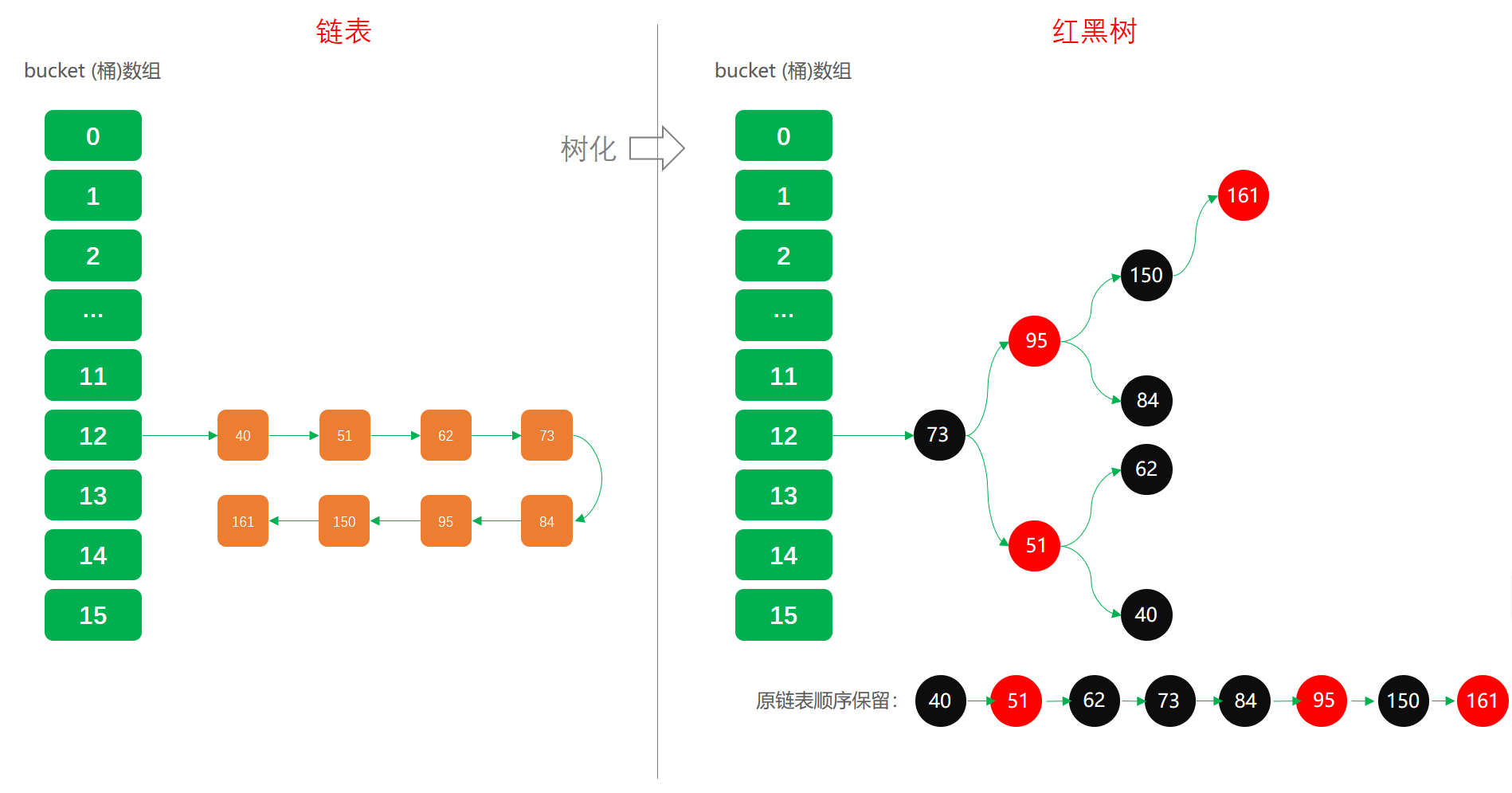``````final void treeifyBin(Node<K,V>[] tab, int hash) {
int n, index; Node<K,V> e;
// 这块就是我们上面提到的，不一定树化还可能只是扩容。主要桶数组容量是否小于64 MIN_TREEIFY_CAPACITY
if (tab == null || (n = tab.length) < MIN_TREEIFY_CAPACITY)
resize();
else if ((e = tab[index = (n - 1) & hash]) != null) {
// 又是单词缩写；hd = head (头部)，tl = tile (结尾)
TreeNode<K,V> hd = null, tl = null;
do {
// 将普通节点转换为树节点，但此时还不是红黑树，也就是说还不一定平衡
TreeNode<K,V> p = replacementTreeNode(e, null);
if (tl == null)
hd = p;
else {
p.prev = tl;
tl.next = p;
}
tl = p;
} while ((e = e.next) != null);
if ((tab[index] = hd) != null)
// 转红黑树操作，这里需要循环比较，染色、旋转。关于红黑树，在下一章节详细讲解
hd.treeify(tab);
}
}``````

1. 链表树化的条件有两点；链表长度大于等于8、桶容量大于64，否则只是扩容，不会树化。
2. 链表树化的过程中是先由链表转换为树节点，此时的树可能不是一颗平衡树。同时在树转换过程中会记录链表的顺序，`tl.next = p`，这主要方便后续树转链表和拆分更方便。
3. 链表转换成树完成后，在进行红黑树的转换。先简单介绍下，红黑树的转换需要染色和旋转，以及比对大小。在比较元素的大小中，有一个比较有意思的方法，`tieBreakOrder`加时赛，这主要是因为HashMap没有像TreeMap那样本身就有Comparator的实现。

#### 1.5 红黑树转链

``````final Node<K,V> untreeify(HashMap<K,V> map) {
Node<K,V> hd = null, tl = null;
// 遍历TreeNode
for (Node<K,V> q = this; q != null; q = q.next) {
// TreeNode替换Node
Node<K,V> p = map.replacementNode(q, null);
if (tl == null)
hd = p;
else
tl.next = p;
tl = p;
}
return hd;
}

// 替换方法
Node<K,V> replacementNode(Node<K,V> p, Node<K,V> next) {
return new Node<>(p.hash, p.key, p.value, next);
}``````

### 2. 查找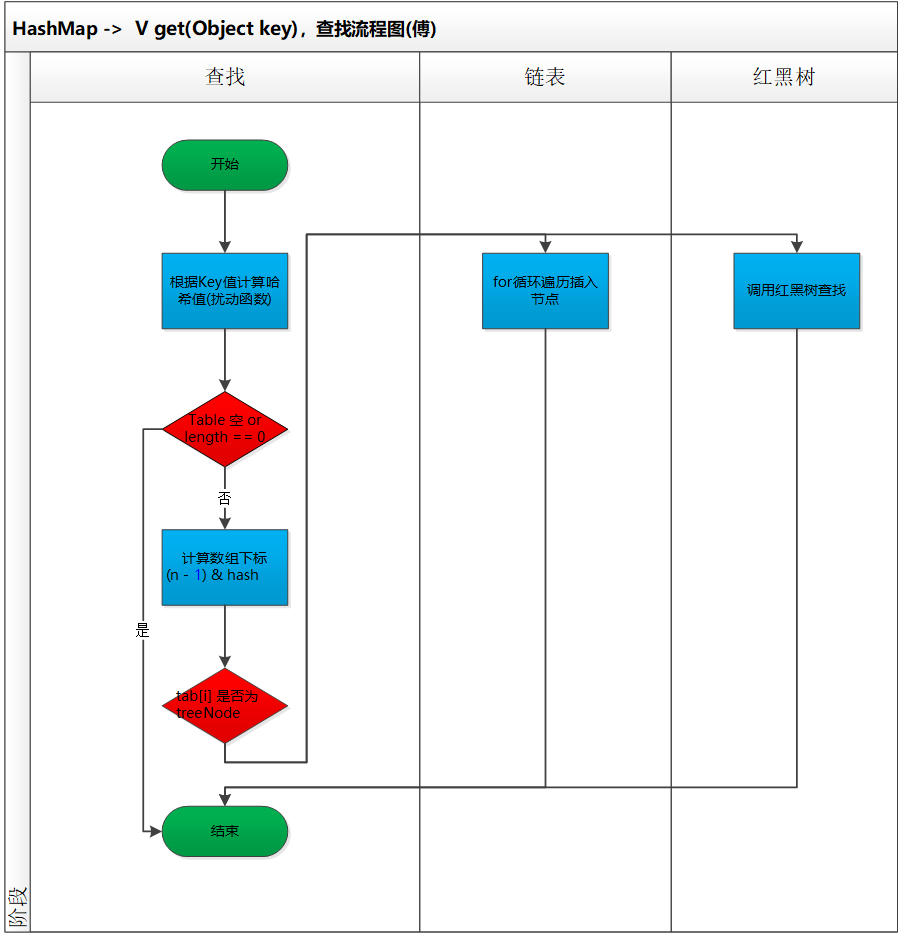``````public V get(Object key) {
Node<K,V> e;
// 同样需要经过扰动函数计算哈希值
return (e = getNode(hash(key), key)) == null ? null : e.value;
}

final Node<K,V> getNode(int hash, Object key) {
Node<K,V>[] tab; Node<K,V> first, e; int n; K k;
// 判断桶数组的是否为空和长度值
if ((tab = table) != null && (n = tab.length) > 0 &&
// 计算下标，哈希值与数组长度-1
(first = tab[(n - 1) & hash]) != null) {
if (first.hash == hash && // always check first node
((k = first.key) == key || (key != null && key.equals(k))))
return first;
if ((e = first.next) != null) {
// TreeNode 节点直接调用红黑树的查找方法，时间复杂度O(logn)
if (first instanceof TreeNode)
return ((TreeNode<K,V>)first).getTreeNode(hash, key);
// 如果是链表就依次遍历查找
do {
if (e.hash == hash &&
((k = e.key) == key || (key != null && key.equals(k))))
return e;
} while ((e = e.next) != null);
}
}
return null;
}``````

1. 扰动函数的使用，获取新的哈希值，这在上一章节已经讲过
2. 下标的计算，同样也介绍过 `tab[(n - 1) & hash])`
3. 确定了桶数组下标位置，接下来就是对红黑树和链表进行查找和遍历操作了

### 3. 删除

`````` public V remove(Object key) {
Node<K,V> e;
return (e = removeNode(hash(key), key, null, false, true)) == null ?
null : e.value;
}

final Node<K,V> removeNode(int hash, Object key, Object value,
boolean matchValue, boolean movable) {
Node<K,V>[] tab; Node<K,V> p; int n, index;
// 定位桶数组中的下标位置，index = (n - 1) & hash
if ((tab = table) != null && (n = tab.length) > 0 &&
(p = tab[index = (n - 1) & hash]) != null) {
Node<K,V> node = null, e; K k; V v;
// 如果键的值与链表第一个节点相等，则将 node 指向该节点
if (p.hash == hash &&
((k = p.key) == key || (key != null && key.equals(k))))
node = p;
else if ((e = p.next) != null) {
// 树节点，调用红黑树的查找方法，定位节点。
if (p instanceof TreeNode)
node = ((TreeNode<K,V>)p).getTreeNode(hash, key);
else {
// 遍历链表，找到待删除节点
do {
if (e.hash == hash &&
((k = e.key) == key ||
(key != null && key.equals(k)))) {
node = e;
break;
}
p = e;
} while ((e = e.next) != null);
}
}

// 删除节点，以及红黑树需要修复，因为删除后会破坏平衡性。链表的删除更加简单。
if (node != null && (!matchValue || (v = node.value) == value ||
(value != null && value.equals(v)))) {
if (node instanceof TreeNode)
((TreeNode<K,V>)node).removeTreeNode(this, tab, movable);
else if (node == p)
tab[index] = node.next;
else
p.next = node.next;
++modCount;
--size;
afterNodeRemoval(node);
return node;
}
}
return null;
} ``````
• 删除的操作也比较简单，这里面都没有太多的复杂的逻辑。
• 另外红黑树的操作因为被包装了，只看使用上也是很容易。

### 4. 遍历

#### 4.1 问题点

HashMap中的遍历也是非常常用的API方法，包括；

KeySet

`````` for (String key : map.keySet()) {
System.out.print(key + " ");
}``````

EntrySet

`````` for (HashMap.Entry entry : map.entrySet()) {
System.out.print(entry + " ");
}``````

#### 4.2 用代码测试

1. 这里我们要设定一个既有红黑树又有链表结构的数据场景
2. 为了可以有这样的数据结构，我们最好把HashMap的初始长度设定为64，避免在链表超过8位后扩容，而是直接让其转换为红黑树。
3. 找到18个元素，分别放在不同节点(这些数据通过程序计算得来)；
1. 桶数组02节点：24、46、68
2. 桶数组07节点：29
3. 桶数组12节点：150、172、194、271、293、370、392、491、590

``````@Test
public void test_Iterator() {
Map<String, String> map = new HashMap<String, String>(64);
map.put("24", "Idx：2");
map.put("46", "Idx：2");
map.put("68", "Idx：2");
map.put("29", "Idx：7");
map.put("150", "Idx：12");
map.put("172", "Idx：12");
map.put("194", "Idx：12");
map.put("271", "Idx：12");
System.out.println("排序01：");
for (String key : map.keySet()) {
System.out.print(key + " ");
}

map.put("293", "Idx：12");
map.put("370", "Idx：12");
map.put("392", "Idx：12");
map.put("491", "Idx：12");
map.put("590", "Idx：12");
System.out.println("\n\n排序02：");
for (String key : map.keySet()) {
System.out.print(key + " ");
}

map.remove("293");
map.remove("370");
map.remove("392");
map.remove("491");
map.remove("590");
System.out.println("\n\n排序03：");
for (String key : map.keySet()) {
System.out.print(key + " ");
}

}``````

1. 添加元素，在HashMap还是只链表结构时，输出测试结果01
2. 添加元素，在HashMap转换为红黑树时候，输出测试结果02
3. 删除元素，在HashMap转换为链表结构时，输出测试结果03

#### 4.3 测试结果分析

``````排序01：
24 46 68 29 150 172 194 271

24 46 68 29 271 150 172 194 293 370 392 491 590

24 46 68 29 172 271 150 194
Process finished with exit code 0``````

1. 01情况下，排序定位哈希值下标和链表信息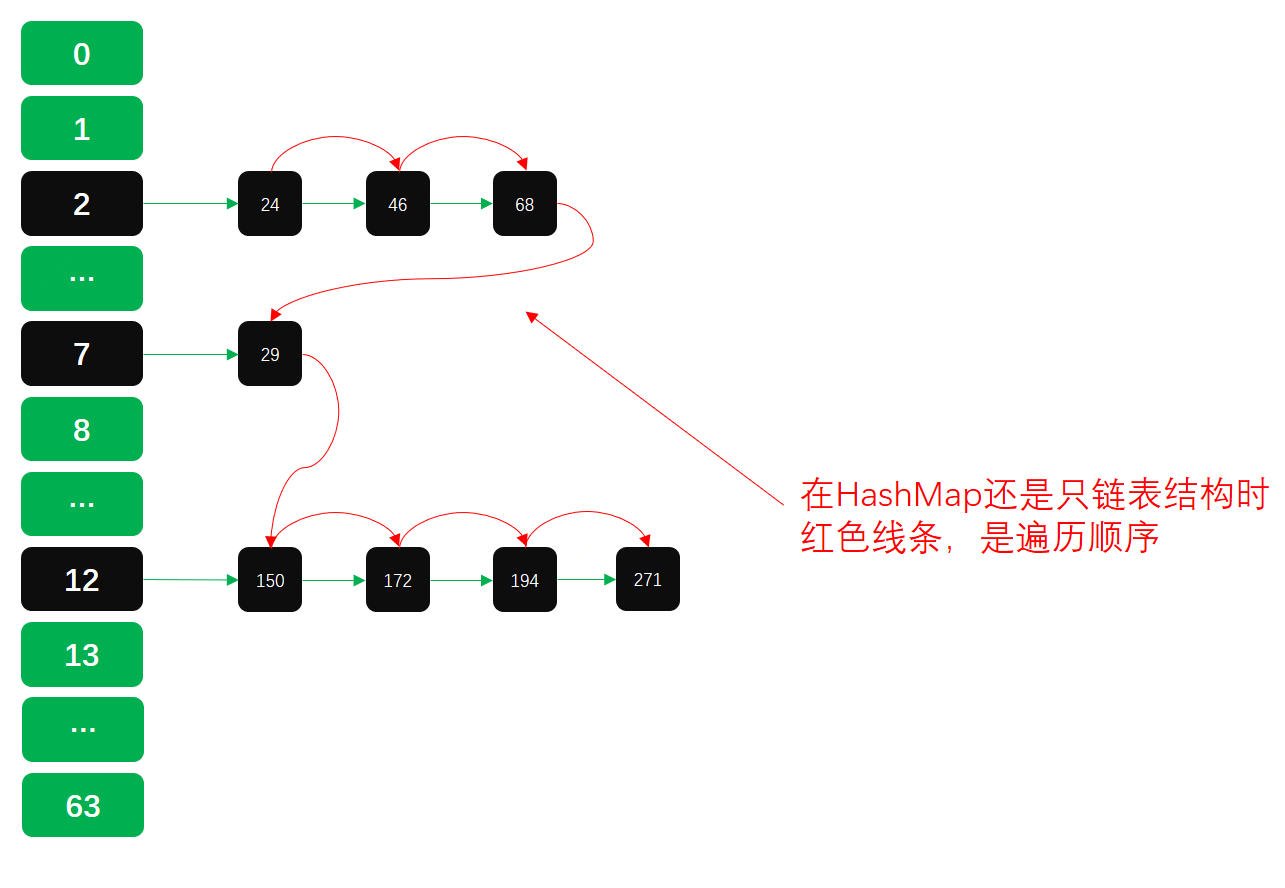1. 02情况下，因为链表转换为红黑树，树根会移动到数组头部。`moveRootToFront()方法`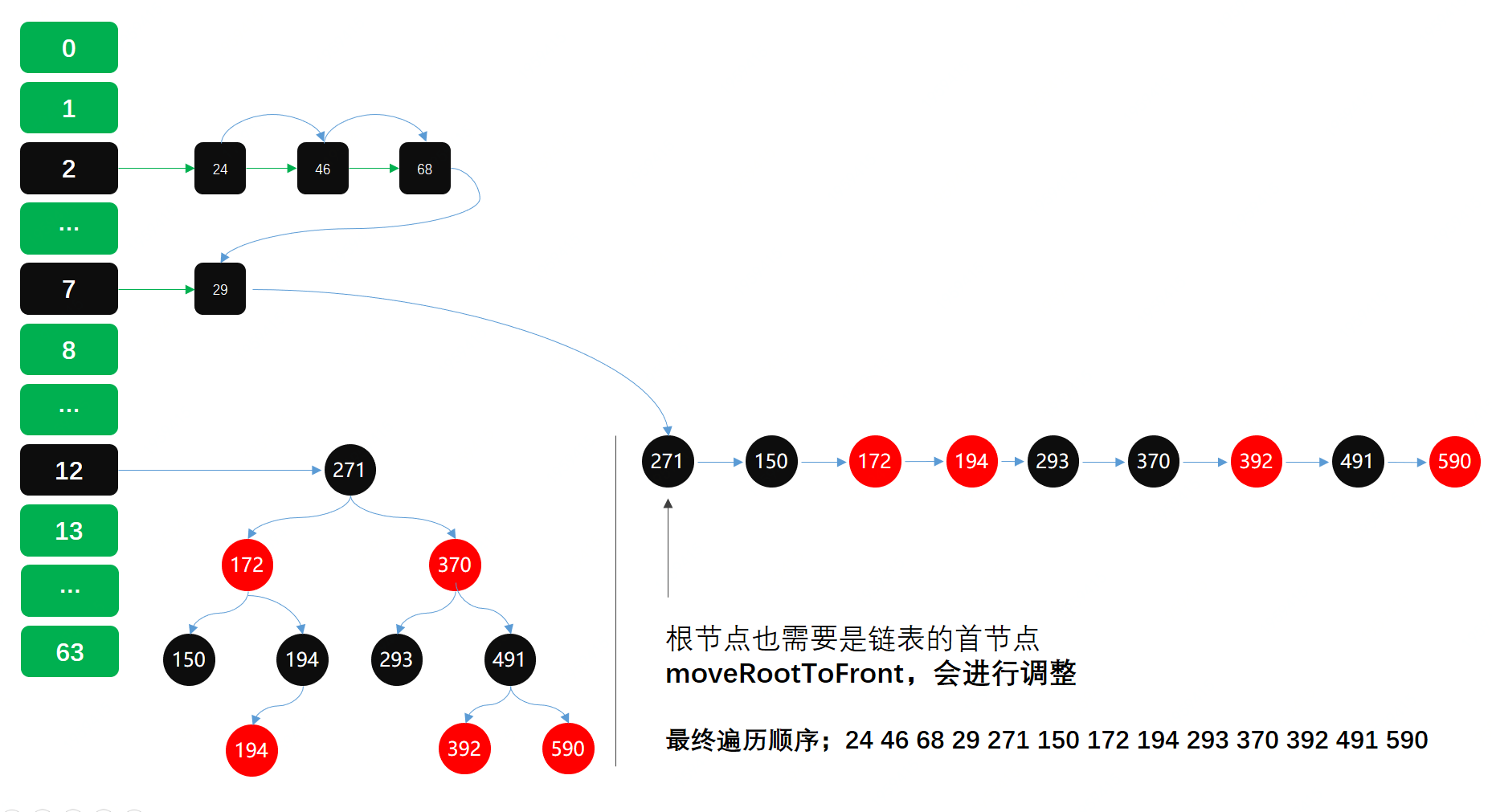1. 03情况下，因为删除了部分元素，红黑树退化成链表。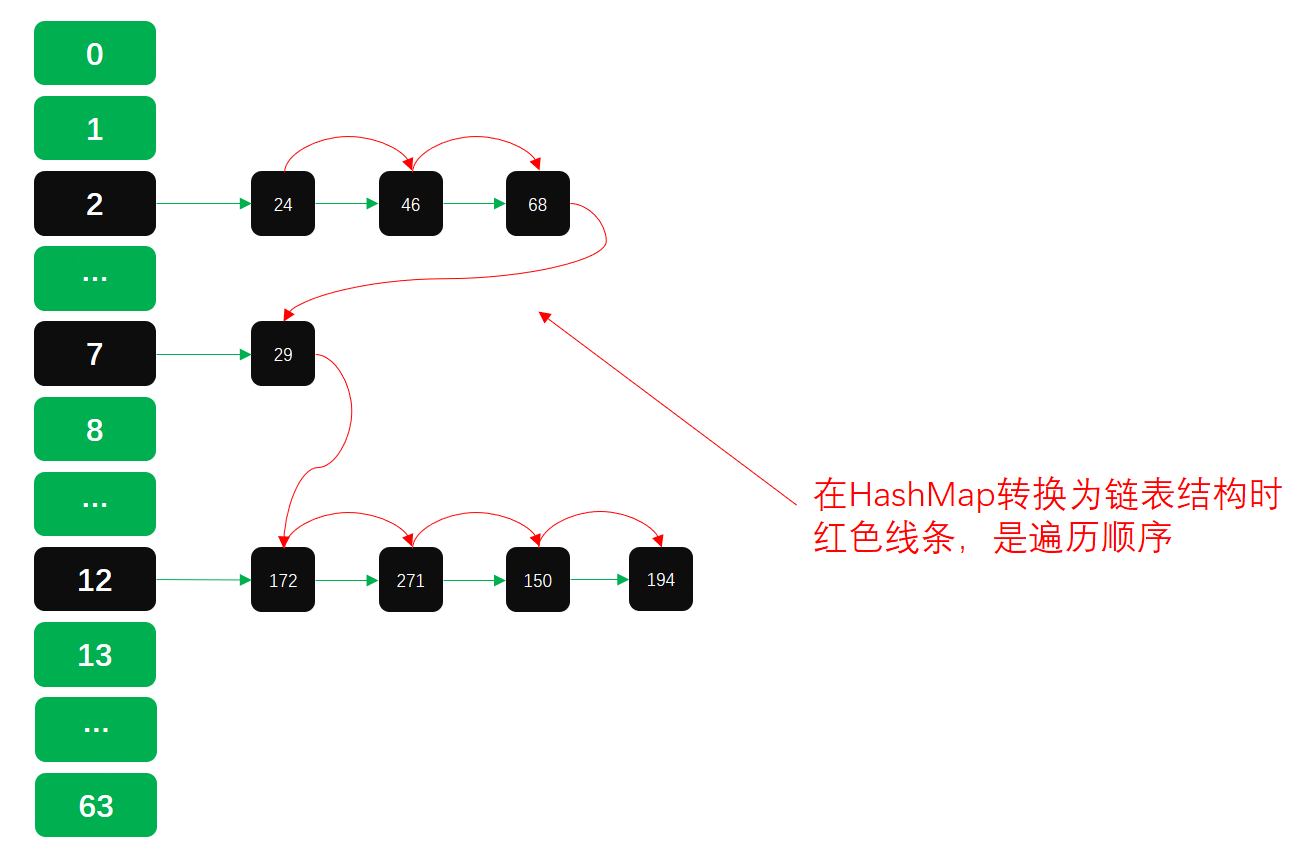## 三、总结

• 这一篇API源码以及逻辑与上一篇数据结构中扰动函数、负载因子、散列表实现等，内容的结合，算是把HashMap基本常用技术点，梳理完成了。但知识绝不止于此，这里还有红黑树的相关技术内容，后续会进行详细。
• 除了HashMap以外还有TreeMap、ConcurrentHashMap等，每一个核心类都有一些相关的核心知识点，每一个都非常值得深入研究。这个烧脑的过程，是学习获得知识的最佳方式。
• 可能关于HashMap还有一些疏漏的点，也希望阅读的小伙伴可以提出更多的问题，互相学习，共同进步，本文就到这里，感谢您的阅读！

## 四、推荐阅读Lv1

9

8

0